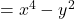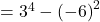## 1 Type the correct answer in the box. Use numerals instead of words. Consider this expression. x4 – y2 When x = 3 and Y = -6 the value of th

Question

1 Type the correct answer in the box. Use numerals instead of words. Consider this expression. x4 – y2 When x = 3 and Y = -6 the value of the expression is ​

in progress 0
2 months 2021-07-23T13:43:25+00:00 1 Answers 5 views 0

1. Step-by-step explanation:

Hey there!

Given expression is;The values of x and y is 3 and -6 respectively.

Put their values.= 81 36